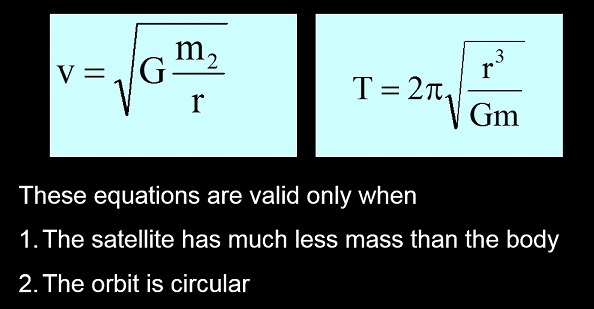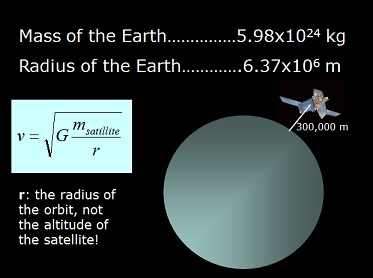# Going Full Circle on Gravity and Orbits - Day 1

6 teachers like this lesson
Print Lesson

## Objective

Students apply the circular motion equations to Newton's Universal Law of Gravity to derive circular orbit equations.

#### Big Idea

The equations for period and velocity for a satellite moving in a circular orbit can be derived by combining Newton's Universal Law of Gravity and the centripetal force equations.

## Context

Much like in the lesson on Projectile Prediction, this lesson has students deriving equations based on the situation. In this case, they derive the equations for the velocity and period of a satellite in a circular orbit.The derivation requires Newton's gravity equation learned last lesson, The First Universal Law, and formula from Playing "A-Round" with Circular Motion. The equations allow students to calculate the orbital velocity and orbital period in terms of the mass of the large body being orbited, the radius of the orbit and the gravitational constant.

This involves CCSS Math Practice 4: Model with mathematics and NGSS Science Practice 5 Using mathematics and computational thinking. In order to perform this analysis, students will have to ask the right questions which is Science Practice 1: Asking questions (for science) and defining problems (for engineering).  There are two NGSS performance standards which are in use for this activity, HS-PS2-4: Use mathematical representations of Newton’s Law of Gravitation Law to describe and predict the gravitational forces between objects and from HS.Space Systems, HS-ESS1-4: Use mathematical or computational representations to predict the motion of orbiting objects in the solar system.

## Deriving the Orbital Equations

10 minutes

I tell students that, "today you are NASA scientists". Their job is to determine the orbital conditions for a new satellite that will monitor the polar ice caps. In order to accomplish this feat of engineering, they apply Newton's Universal Law of Gravity to an object in circular orbit. The first slide of the Orbital period and velocity power point is displayed on the board as I give this overview.

The next few slides are review of the law of gravity learned last lesson, The First Universal Law. I expect students to write down the problems and practice them in their notebooks on their own. After students work through the problems, the answers are displayed in the power point. (Please note that animations in the power point do not work correctly in the preview mode; for full functionality, download the power point file.)

Then, as a class, we derive the formulas for velocity and period of a satellite in a circular orbit. I communicate that the students are expected to write down each step, as they need to reproduce these steps in the future. The power point leads the students through the process step-by-step. We begin with the centripetal force equation and set it equal to the law of gravity, since it is gravity that is applying the force that keeps a satellite in orbit. The hope in this exercise is that students understand that modeling with math is a powerful tool with many applications.

## Mission Control Needs YOU!

15 minutes

I pretend to take a phone call on my smart phone, saying "Yes sir, right away sir. Oh yes, my scientist students are up to the challenge!".  Then, I tell the students that I just talked to the NASA Director and that he wants their help! I display the first slide of Mission Control Needs You power point.

The next few slides details their mission, which is to determine the orbital conditions (velocity, period and radius) for a new satellite. The satellite is to take high resolution pictures in order to monitor the snow and ice covering over the arctic and antarctic. This requires that it must be at an altitude of 300 kilometers. Then I reveal a most vital part of the mission... the satellite name. NASA loves acronyms (I worked on the ACE satellite when I was in college which I show on the power point). I ask students to make an acronym for this mission that is both descriptive and a word. I provide some of the more fun and create examples from past years "mission scientists".

I display the requirements for this mission (slide 6) which details that the students must determine a name for the new satellite as well as the orbital velocity, period and radius given the requirement that it is 300 km above the Earth. Students can commence with the mission only after they prove derive the orbital equations on their own. All students are given the derive orbital equations sheet where they reproduce the orbital equations derivation, but they must do it independently with their notebooks closed.

This is to give them practice deriving equations, an important physics skill. To complete this derivation, they are allowed three life-lines: they can peak in their notebook for 10 seconds, ask a friend a single questions and ask me a single, specific question. Once a student successfully derives the orbital motion equations, they bring their work to me to begin work on the mission.

## Student Scientists Solve Orbital Parameters

25 minutes

As students complete their derivation of orbital period and orbital velocity based on mass and radius, they bring their work to me. As students come up, I check their work and pair up students who have successfully derived the orbital motion equations. Together, the groups of two are to complete the NASA mission of where they determine the name, orbital radius, velocity and period of the new satellite. I continue this way until all students are grouped. This grouping method tends to create homogeneous pairs since the rate at which students finish the derivation is based on their math and physics skills. For this activity, this is appropriate since most students are able to complete this activity with the provided scaffolding.After all students groups are formed, which takes about 10 minutes, I display the final slide from the Mission Control Needs You power point. This slide displays the needed variables for the mission; I spend a minute pointing out how the variables relate to the formulas. For instance, the altitude of the satellite is different from the radius of the orbit. Students have to add the altitude to the radius of the Earth. Also, students need the mass and radius of the Earth (which they should have in their notebooks).  Most of the high performing groups, who have been working on the problem for 10 or more minutes, have figured this out. The groups who are in need of support are just starting the mission when I give this information, so they start with the right numbers.

Then I project the mission details (slide six) for students to reference in their write-up. (The correct answers are also posted in the notes section of slide six.) I intentionally include too much information on the mission details slide. I do this so that students have to choose what information is needed and what can be ignored. It is good to do this as it mirrors reality. Rarely, when faced with a complex task, do scientists have only the essential information. They often have to sift through a irrelevant information to find what information is needed.

As the lesson time concludes, I collect the student sheets to assess their understanding and assign a grade to this activity. I inform the students that tomorrow, they are to continue their roles as NASA scientists as they again apply the orbital equations to determine satellite positions.